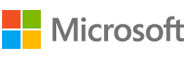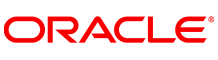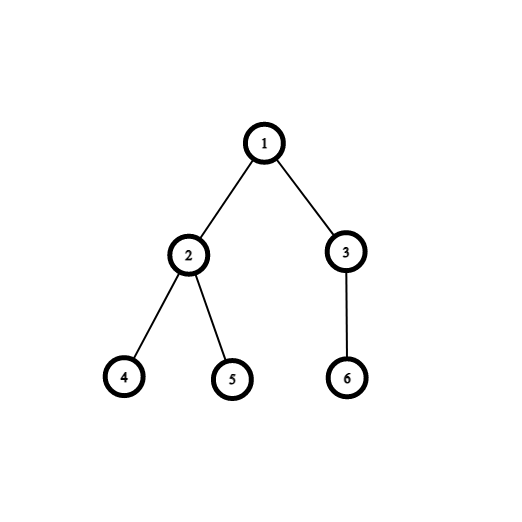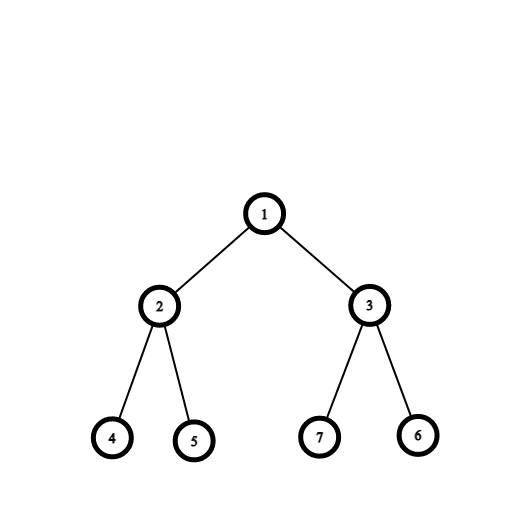New update is available. Click here to update.
Topics

# Nodes In Complete Binary Tree

Moderate0/80
Average time to solve is 15m+5 more companies

## Problem statement

You are given the root of a complete binary tree, you need to calculate the number of nodes in the given complete binary tree.

A complete binary tree is a tree in which all the levels are completely filled except the last level. Nodes in the last level are as left as possible.

For Example:``````In the above complete binary tree, all the levels are filled except for the last. In the last level, all the nodes in the last level are as far left as possible.
``````
Detailed explanation ( Input/output format, Notes, Images )
Sample Input 1:
``````1
1 2 3 4 5 7 6 -1 -1 -1 -1 -1 -1 -1 -1
``````
Sample Output 1:
``````7
``````
Explanation For Sample Input 1:
``````For the above test case, the given tree is:
````````````Level 1 :
The root node of the tree is 1

Level 2 :
Left child of 1 = 2
Right child of 1 = 3

Level 3 :
Left child of 2 = 4
Right child of 2 = 5
Left child of 3 = 7
Right child of 3 = 6

Level 4 :
Left child of 4 = null (-1)
Right child of 4 = null(-1)
Left child of 5 = null (-1)
Right child of 5 = null (-1)
Left child of 7 = null (-1)
Right child of 7 = null (-1).
Left child of 6 = null (-1)
Right child of 6 = null (-1)

Hence the total number of nodes in the given tree is 7.
``````
Sample Input 2:
``````1
5 6 7 10 -1 -1 -1 -1 -1
``````
Sample Output 2:
``````4
``````Console